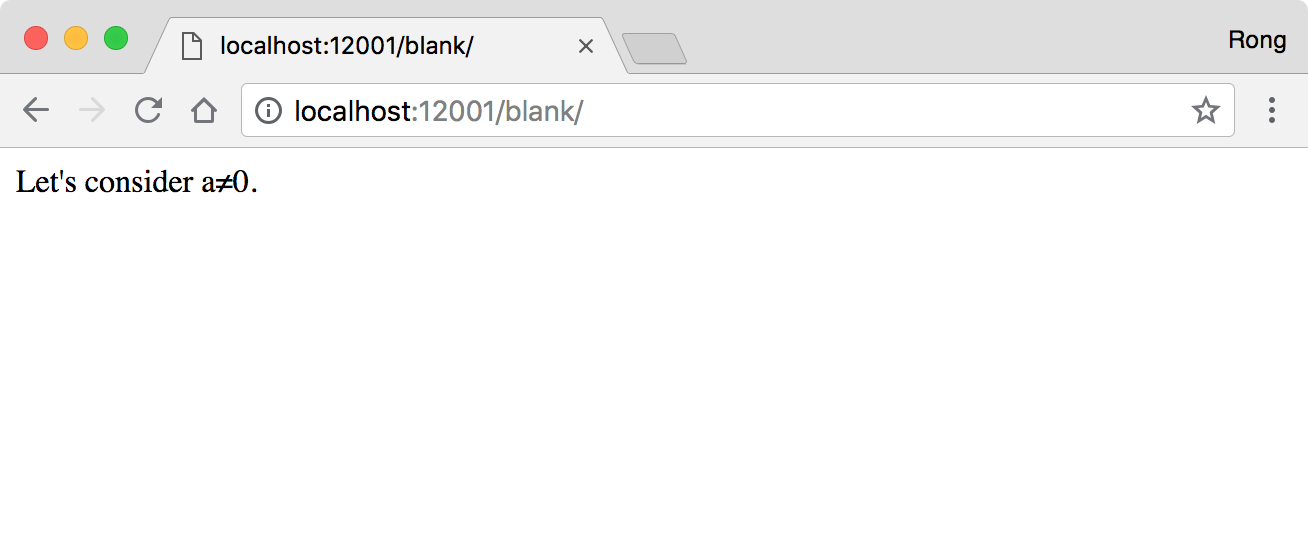Display mathematical notation in web pages with MathJax.

## 1. Math Symbols

### 1. Showing Math Symbols on Web Page

The support of displaying math symbols with html tags is limited. Though you can use UTF-8 to display some special characters, it is hard to remember them and it is inconvenient to use.

Title Formula HTML Tag
Square n2 n<sup>2</sup>
Square Root √, √ &radic;, or &#8730;
Summary ∑, ∑ &sum;, or &#8721;

## 2. Using MathJax on Web Pages

### 2.1 MathJax

MathJax is a cross-browser JavaScript library that displays mathematical notation in web browsers.

The preferred way to use MathJax on a web page is by linking to the publicly available MathJax Content Delivery Network(CDN). This can be done by adding the following code snippet into the HTML header block(the code between <head> and </head>) of your HTML or XHTML document:

<script type="text/javascript"
src="http://cdn.mathjax.org/mathjax/latest/MathJax.js?config=TeX-AMS-MML_HTMLorMML">
</script>


For greater security, it is also possible to access the CDN via https:

<script type="text/javascript" async
src="https://cdnjs.cloudflare.com/ajax/libs/mathjax/2.7.5/latest.js?config=TeX-MML-AM_CHTML">
</script>


### 2.3 Including MathML

With the link to MathJax in the HTML header in place, MathJax will recognize and render MathML elements that are included in the document with the standard $ tags. A minimal example of a fully functional HTML page with embedded MathML using MathJax from the CDN could be the following: <!DOCTYPE html> <html> <head> <script type="text/javascript" src="http://cdn.mathjax.org/mathjax/latest/MathJax.js?config=TeX-AMS-MML_HTMLorMML"> </script> </head> <body> Let's consider [itex]<mi>a</mi><mo>≠</mo><mn>0</mn>$.
</body>
</html>## 3. Using MathJax in Jekyll with Markdown

### 3.1 Page Template

Create a file named with mathjax.html in _include directory as follows:

<script type="text/x-mathjax-config">
MathJax.Hub.Config({
TeX: {
equationNumbers: {
autoNumber: "AMS"
}
},
tex2jax: {
inlineMath: [ ['$','$'], ['\$$', '\$$'] ],
displayMath: [ ['$$','$$'] ],
processEscapes: true,
}
});
</script>
<script type="text/javascript" src="http://cdn.mathjax.org/mathjax/latest/MathJax.js?config=TeX-AMS-MML_HTMLorMML"></script>

• See the details about the settings from here, especially for the escape character $and (). Update file _inlude/head.html, include the above template file if page.mathjax is true. {% if page.mathjax %} {% include mathjax.html %} {% endif %}  ### 3.2 Using Math Formula in Markdown Page In order to use the math formula in markdown page, it must have a variable mathjax and set its value to true. For example, the following example is a markdown page using mathjax. --- title: "Using MathJax in Jekyll" date: 2018-07-10 tags: [MathJax, Mathematic] mathjax: true ---  1) Example One: Construct the formula with following markdown. $a^2 + b^2 = c^2$ Then you will get the formula as follows.$a^2 + b^2 = c^2$2) Example Two: Construct the formula with following markdown. $ x = {-b \pm \sqrt{b^2-4ac} \over 2a} $ Then you will get the formula as follows.$ x = {-b \pm \sqrt{b^2-4ac} \over 2a} $3) Example Three: Construct the formula with following markdown. $$\begin{eqnarray} x' &=& &x \sin\phi &+& z \cos\phi \\ z' &=& - &x \cos\phi &+& z \sin\phi \\ \end{eqnarray}$$  Then you will get the formula as follows. $\begin{eqnarray} x' &=& &x \sin\phi &+& z \cos\phi \\ z' &=& - &x \cos\phi &+& z \sin\phi \\ \end{eqnarray}$ Here are some notes about the above example: • The inline formula is between $ ... \$.
• The display formula is between $$...$$.
• You can use the math envrionment directly, for example, \begin{equation}...\end{equation} or \begin{align}...\end{align}.
• Whenever in the inline math or display math, the star character * must be escaped.
• In the multi-lines display math, the line break symbol double-backslash \\ should be escaped, i.e., use four backslash \\\\.
• If you found error while typesetting math formula, try to escape some special characters.